# Chemistry Question

Calculate the mass of ice required to cool  of beverage from  to . Assume a density of 1.00 g/mL and a specific heat capacity of  for the beverage. The enthalpy of fusion of ice is

We need at least 9 more requests to produce the answer.

1 / 10 have requested this problem solution

The more requests, the faster the answer.

All students who have requested the answer will be notified once they are available.

#### Earn Coin

Coins can be redeemed for fabulous gifts.

Similar Homework Help Questions
• ### An ice cube with a mass of 46.4 g at 0.0 ∘C is added to a...

An ice cube with a mass of 46.4 g at 0.0 ∘C is added to a glass containing 4.20×102 g of water at 45.0 ∘C . Determine the final temperature of the system at equilibrium. The specific heat capacity of water, ?s , is 4.184 J/g⋅∘C , and the standard enthalpy of fusion, Δ?∘fus , of water is 6.01×103 J/mol . Assume that no energy is transferred to or from the surroundings.

• ### An ice cube with a mass of 53.0 g at 0.0 °C is added to a...

An ice cube with a mass of 53.0 g at 0.0 °C is added to a glass containing 368 g of water at 45.0 °C. Determine the final temperature of the system at equilibrium. The specific heat capacity of water, Cs, is 4.184 J/g·°C and the standard enthalpy of fusion, ΔH°fus, of water is 6.01 × 103 J/mol. Assume that no energy is transferred to or from the surroundings.

• ### 10) How many grams of ice do I need to add to the 356 grams of...

10) How many grams of ice do I need to add to the 356 grams of my soda if I wish to lower the temperature of my soda from 21.8°C to 3.9°C. Assume that the specific heat of the soda is 4.172 J/g⋅C°. The specific heat of water is 4.184 J/g⋅C°. The heat capacity of the glass is 56.4 J/C°. The molar enthalpy of fusion (melting) of ice is 6.018 kJ/mol.

• ### How much heat is released when 105 g of steam at 100.0°C is cooled to ice...

How much heat is released when 105 g of steam at 100.0°C is cooled to ice at -15.0°C? The enthalpy of vaporization of water is 40.67 kJ/mol, the enthalpy of fusion for water is 6.01 kJ/mol, the molar heat capacity of liquid water is 75.4 J/(mol • °C), and the molar heat capacity of ice is 36.4 J/(mol • °C). A)347 kJ B)54.8 kJ C)319 kJ D)273 kJ

• ### C) 3400 D ) 2000 Q10: 1.0 L of 1 M NaOH(aq) and 1.0 L of...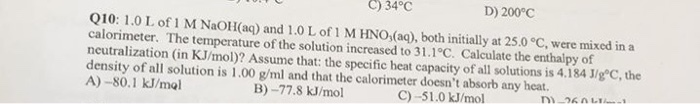C) 3400 D ) 2000 Q10: 1.0 L of 1 M NaOH(aq) and 1.0 L of 1 M HNO3(aq), both initially at 25.0 °C, were mixed in a calorimeter. The temperature of the solution increased to 31.1°C. Calculate the enthalpy of neutralization in KJ/mol)? Assume that the specific heat capacity of all solutions is 4.184 J/gºC, the density of all solution is 1.00 g/ml and that the calorimeter doesn't absorb any heat. A) -80.1 kJ/mol B) - 77.8 kJ/mol C)...

• ### Enter your answer in the provided box. How much heat (in kJ) is needed to convert...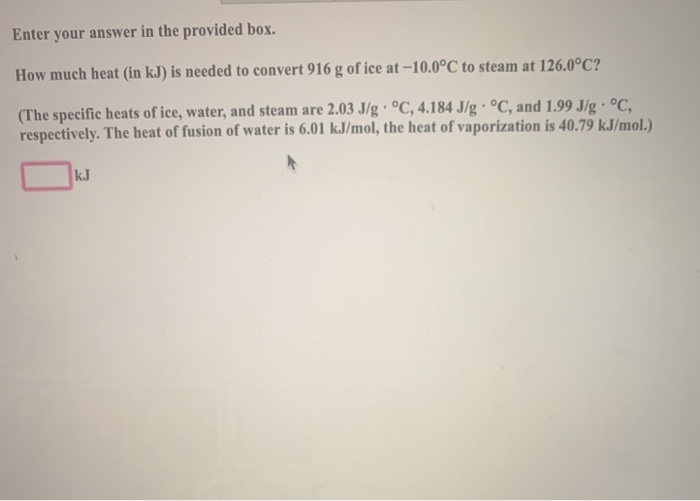Enter your answer in the provided box. How much heat (in kJ) is needed to convert 916 g of ice at -10.0°C to steam at 126.0°C? (The specific heats of ice, water, and steam are 2.03 J/g . oC, 4.184 J/g . oC, and 1.99 J/g , oC, respectively. The heat of fusion of water is 6.01 kJ/mol, the heat of vaporization is 40.79 k.J/mol.) k.J

• ### Two 20.0 g ice cubes at -12.0 °C are placed into 215 g of water at...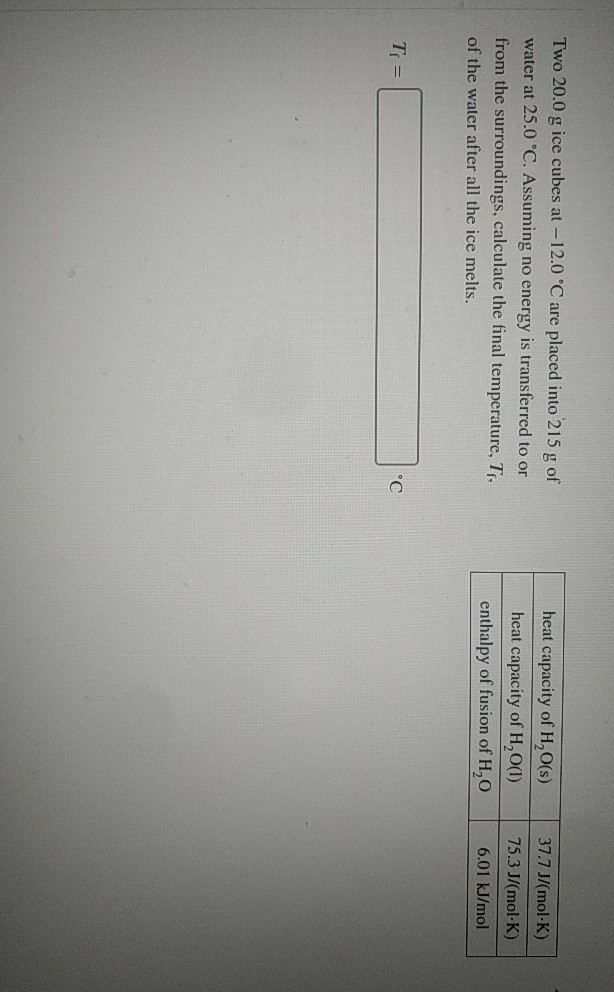Two 20.0 g ice cubes at -12.0 °C are placed into 215 g of water at 25.0 °C. Assuming no energy is transferred to or from the surroundings, calculate the final temperature, T, of the water after all the ice melts. heat capacity of H2O(s) heat capacity of H2O(1) enthalpy of fusion of H,O 37.7 J/(mol-K) 75.3 J/(mol-K) 6.01 kJ/mol Ti =

• ### You order a 16 oz glass of tea (where the mass of water is 474 grams)...

You order a 16 oz glass of tea (where the mass of water is 474 grams) from a local restaurant. The tea is freshly brewed and has an initial temperature of 20.73 °C. You add ice to cool it. If the heat of fusion of ice is 6.020 kJ/mol and each ice cube contains exactly 1 mol of water (assume the ice does not add anymore volume), how many ice cubes are necessary to cool the tea to 0.240 °C?...

• ### Please answer and show work and explain 10. 100.0 mL of 0.600M HCl is reacted with...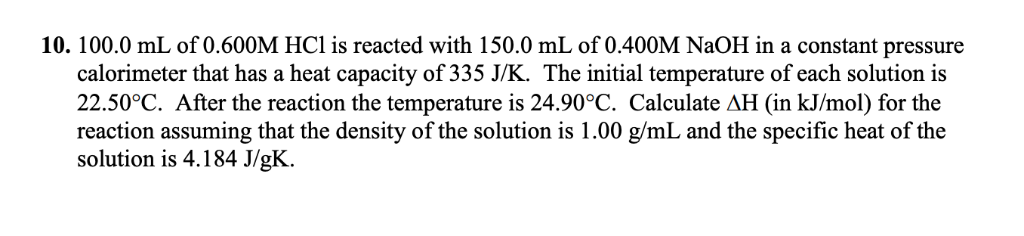Please answer and show work and explain 10. 100.0 mL of 0.600M HCl is reacted with 150.0 mL of0.400M N2OH in a constant pressure calorimeter that has a heat capacity of 335 J/K. The initial temperature of each solution is 22.50°C. After the reaction the temperature is 24.90°C. Calculate AH (in kJ/mol) for the reaction assuming that the density of the solution is 1.00 g/mL and the specific heat of the solution is 4.184 J/gK 10. 100.0 mL of 0.600M...

• ### Two 20.0 g ice cubes at -12.0 °C are placed into 225 g of water at...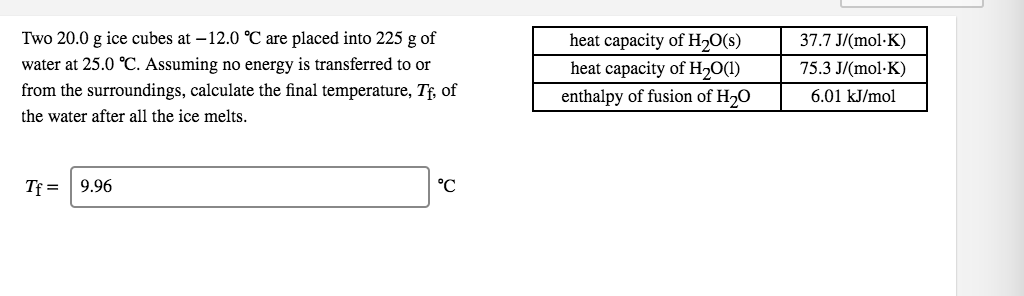Two 20.0 g ice cubes at -12.0 °C are placed into 225 g of water at 25.0 °C. Assuming no energy is transferred to or from the surroundings, calculate the final temperature, Tf, of the water after all the ice melts. heat capacity of H2O(s) heat capacity of H2O(1) enthalpy of fusion of H20 37.7 J/(molK) 75.3 J/(mol·K) 6.01 kJ/mol Tf= 9.96